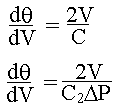### Filtration - Constant Pressure & Constant Rate Operation

A filtration is carried out for 10 min at a constant rate in a leaf filter and thereafter it is continued at constant pressure. This pressure is that attained at the end of the constant rate period. If one quarter of the total volume of the filtrate is collected during the constant rate period, what is the total filtration time? Assume that the cake is incompressible and the filter medium resistance is negligible.

Calculations:

For filtration operation,à 1

where C is a function of DP and mean specific cake resistance (a).

i.e., C = C1DP/a à 2

C1 is a constant.

Specific cake resistance a is related to the compressibility coefficient (s) by the relation

a = ao(DP)s

where ao is a constant.

Equn.2 can be written as C = C2DP where C2 is a constant

For an incompressible cake, s = 0; in other words, a is a constant.

If filter medium resistance is negligible, then Vf = 0.

Now the Equn.1 can be written in the form,à 3

For constant rate filtration, dq/dV = Constant = q/V

Given: q = 10 min for V = 0.25 VT

Where VT = total volume of filtrate (filtrate collected in constant rate operation and constant pressure operation)

From Equn.3

2V/(C2DP) = 10/(0.25VT) = 40/VT

i.e., DP = 2V/(40C2/VT) = V VT/(20C2)

DP at the end of constant rate operation = 0.25 VT2 / (20 C2) = 0.0125 VT2/C2

Constant Pressure filtration:

For the given problem conditions C2 = Constant.,

and DP for the constant pressure operation is that obtained at the end of the constant rate operation.

From Equn.3,

dq/dV = 2V/(C2DP) = 2V/(0.0125 VT2) à 4

Integrating Equn.4 between the limits V = 0.25VT to VT and q = 10 min to qT

ò dq = (2/0.0125VT2) ò VdV

qT - 10 = (80/VT2) [VT2 - (0.25VT)2]

qT = 10 + (80/VT2) x 0.9375 x VT2

qT = 10 + 75 = 85 minutes

Total filtration time = 85 minutes

HOME